# Angle Rules Worksheet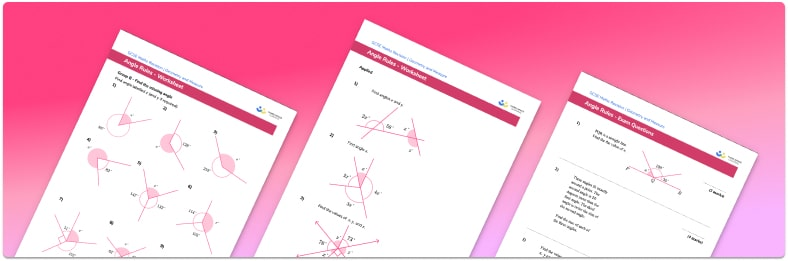• Section 1 of the angles rules worksheet contains 27 skills-based angles rules questions, in 3 groups to support differentiation
• Section 2 contains 3 applied angles rules questions with a mix of worded problems and deeper problem solving questions
• Section 3 contains 6 foundation and higher level GCSE exam style questions
• Answers and a mark scheme for all questions are provided
• Questions follow variation theory with plenty of opportunities for students to work independently at their own level
• All questions created by fully qualified expert secondary maths teachers
• Suitable for GCSE maths revision for AQA, OCR and Edexcel exam boards

• This field is for validation purposes and should be left unchanged.

You can unsubscribe at any time (each email we send will contain an easy way to unsubscribe). To find out more about how we use your data, see our privacy policy.

### Angle rules at a glance

An angle is formed when two line segments or rays intersect at a vertex and are typically measured in degrees using a protractor. We classify angles based on their size. The different types of angle required at GCSE are acute, obtuse, reflex and right angle.

Angle facts are useful for finding missing angles in geometry problems. The basic angle facts used most frequently are: angles in a triangle sum to 180°, angles in a quadrilateral sum to 360°, angles on a straight line sum to 180° and angles around a point sum to 360°.

There are some additional angle properties that are useful when finding unknown angles in 2D shapes. For example, the base angles in an isosceles triangle are equal, as are opposite angles in a parallelogram.

Looking forward, students can then progress to additional geometry worksheets, for example an angles in polygons worksheet or exterior angles worksheet.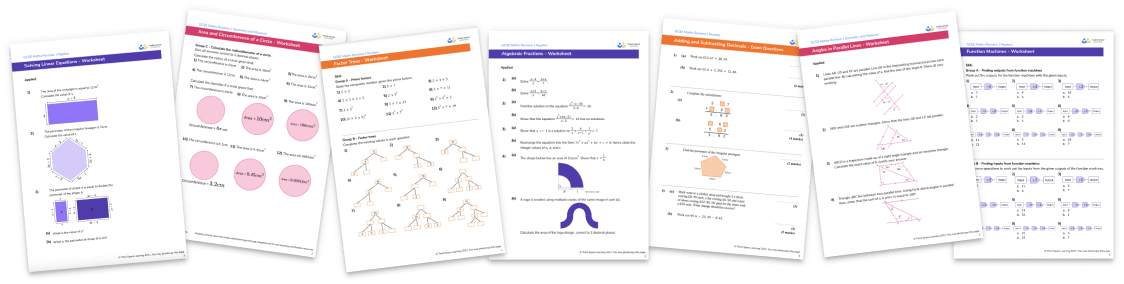For more teaching and learning support on Geometry our GCSE maths lessons provide step by step support for all GCSE maths concepts.

## Related worksheets

Vertically Opposite Angles Worksheet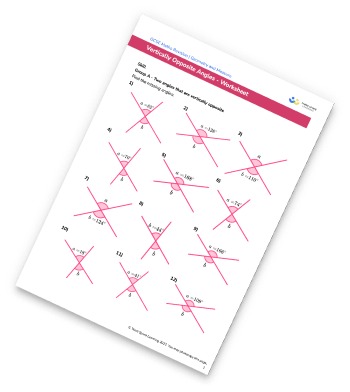Angles Around A Point Worksheet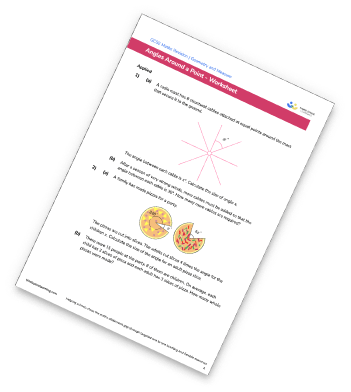Angles On A Straight Line Worksheet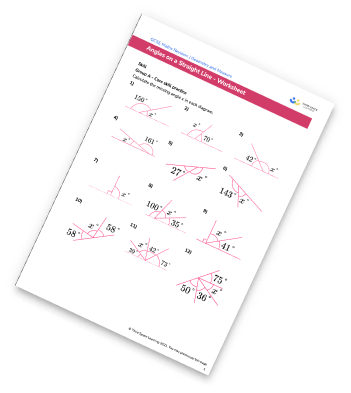Congruent Triangles Worksheet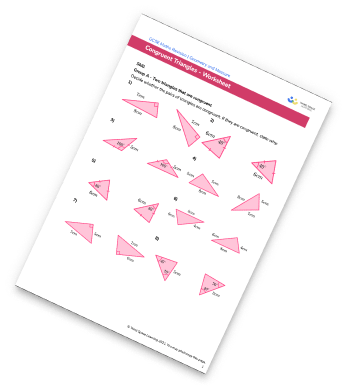## Do you have KS4 students who need more focused attention to succeed at GCSE?There will be students in your class who require individual attention to help them succeed in their maths GCSEs. In a class of 30, it’s not always easy to provide.

Help your students feel confident with exam-style questions and the strategies they’ll need to answer them correctly with our dedicated GCSE maths revision programme.

Lessons are selected to provide support where each student needs it most, and specially-trained GCSE maths tutors adapt the pitch and pace of each lesson. This ensures a personalised revision programme that raises grades and boosts confidence.

Find out more